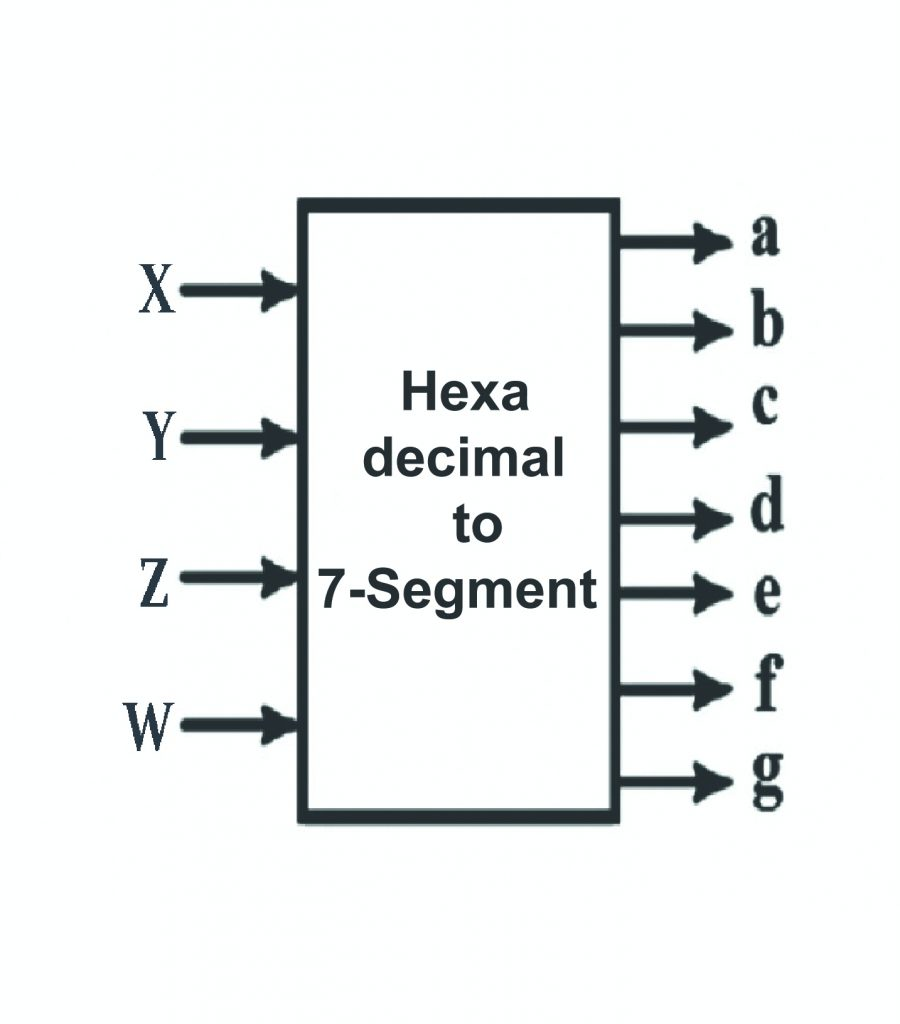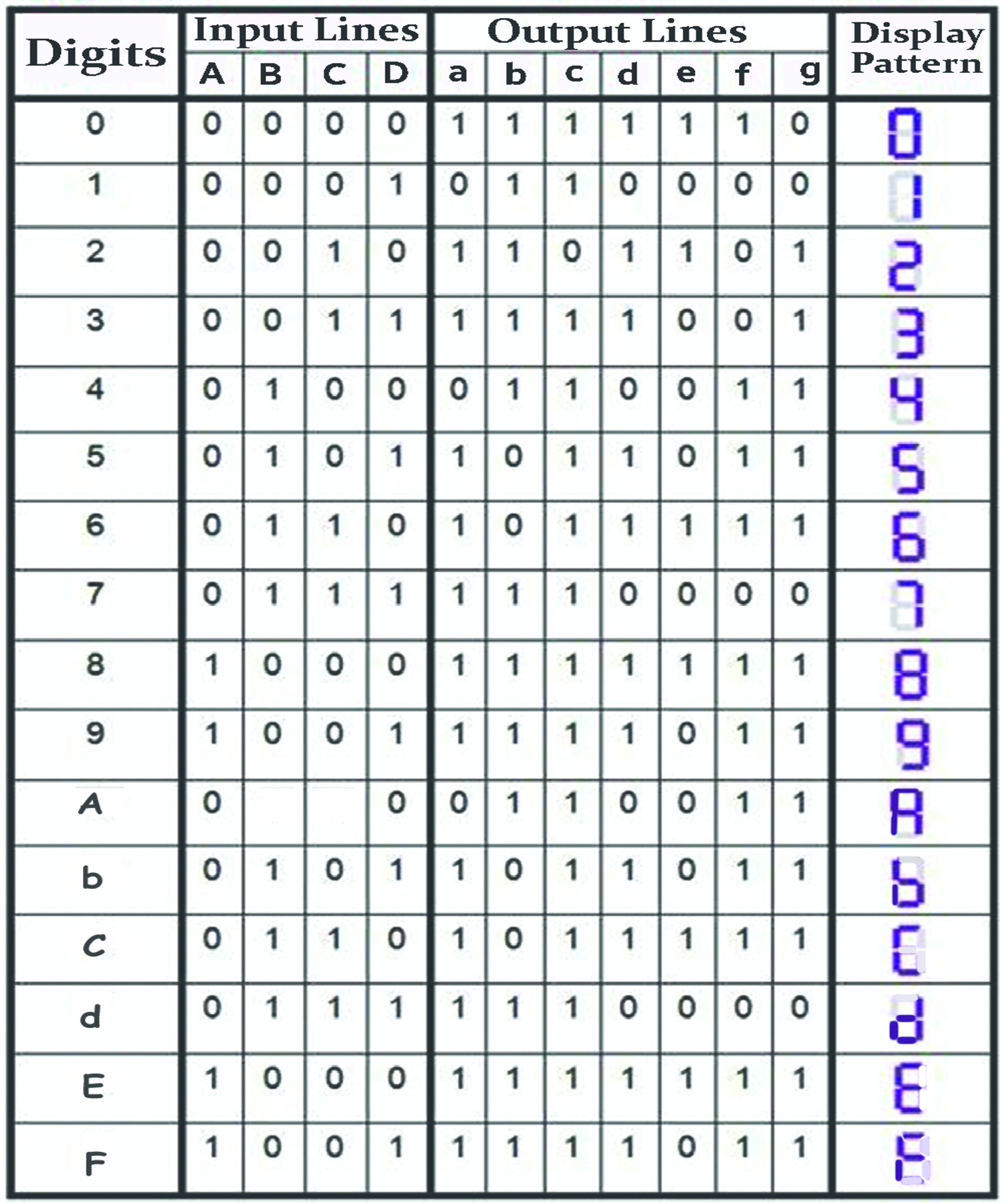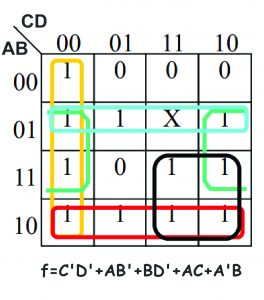# Hexadecimal to Seven segment || Combinational Logic || Bcis Notes

The hexadecimal to seven segment encoder has 4 bit input and 7 output. Depending upon the input number, some of the 7 segments are displayed. The seven segments are represented as a, b, c, d, e, f, g. A high on one of these segments make it display.A combinational circuit that converts a hexadecimal digit to an appropriate code for the selection of segments in an indicator used to display the decimal digit in a familiar form. The seven outputs of the decoder select the corresponding LEDs in the display, as shown in figure below .Truth Table

The truth table for this display is given below:The K Map for a is given below:The K Map for b is given below:The K Map for c is given below:The K Map for d is given below:The K Map for e is given below:The K Map for f is given below:The K Map for g is given below:Therefore these are the detail information related to hexadecimal to 7 segment series with k map of each (a, b, c, d, e, f, g).

You May Also Like: BCD to Seven Segment Display